# Rhombus

Find the length of each side of rhombus if the perimeter is 49 dm long.

a =  12.25 dm

### Step-by-step explanation: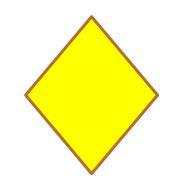We will be pleased if You send us any improvements to this math problem. Thank you!Tips to related online calculators
Do you want to convert length units?

#### You need to know the following knowledge to solve this word math problem:

We encourage you to watch this tutorial video on this math problem:

## Related math problems and questions:

• DiamondThe diamond has a circumference of 48cm. Calculate the length of its side in dm.
• Table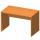Find the circumference of the table, where the long side is 1.28 meters and the short side is 86 cm.
• Triangle ABCIn a triangle ABC side b measure 10 cm less than the side a and side b is half of the side c. Calculate the length of sides if the circumference of the triangle is 42 cm.
• RhombusFind the length of the other diagonal and area of the rhombus. The perimeter of a rhombus is 40 cm and one of the diagonals is of length 10 cm.
• Rhombus problem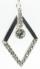Perimeter of the rhombus is 204 dm and it area is 2244 dm2. Calculate the height of the rhombus.
• Rhombus HP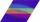Calculate area of the rhombus with height 24 dm and perimeter 12 dm.
• Tablecloth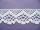The table top is 90 cm long and 50 cm wide. How many cm lace edging is necessary for the tablecloth that hangs on each side by 10 cm?
• RectangleThe length of one side of the rectangle is three times the length of the second side. What are the dimensions of the rectangle if its circumference 96 cm?
• Strip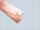From 5.9 cm wide strip should be cut rhombus with area 28 cm2. How long will be its side?
• An equilateral triangle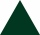The perimeter of an equilateral triangle is 33cm. How long is each side?
• RhombusCalculate the length of the diagonal AC of the rhombus ABCD, if its perimeter is 84 dm and the other diagonal BD has length 20 dm.
• The parallelogram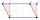The parallelogram has one side 2 dm long, which is one-sixth of its circumference. How many cm does the other side of the parallelogram measure?
• Rhombus sidesfind the sides of a rhombus, it area is 550 cm square and altitude is 55cm
• RectangleThe rectangle area is 182 dm2, its base is 14 dm. How long is the other side? Calculate its perimeter.
• Second sideCalculate the length of the other side of the rectangle if its circumference is 60 cm and one side is 10 cm long.
• RhombusIf we increase the rhombus side by 2.4 cm; its perimeter is 20 cm. How long was the side of the rhombus?
• MO-Z5-3-66 tilesThe picture shows a square tiles with side 10 dm which is composed of four identical small rectangles and squares. Circumference of small square is five times smaller than the circumference of the entire tile. Determine the dimensions of the rectangle.